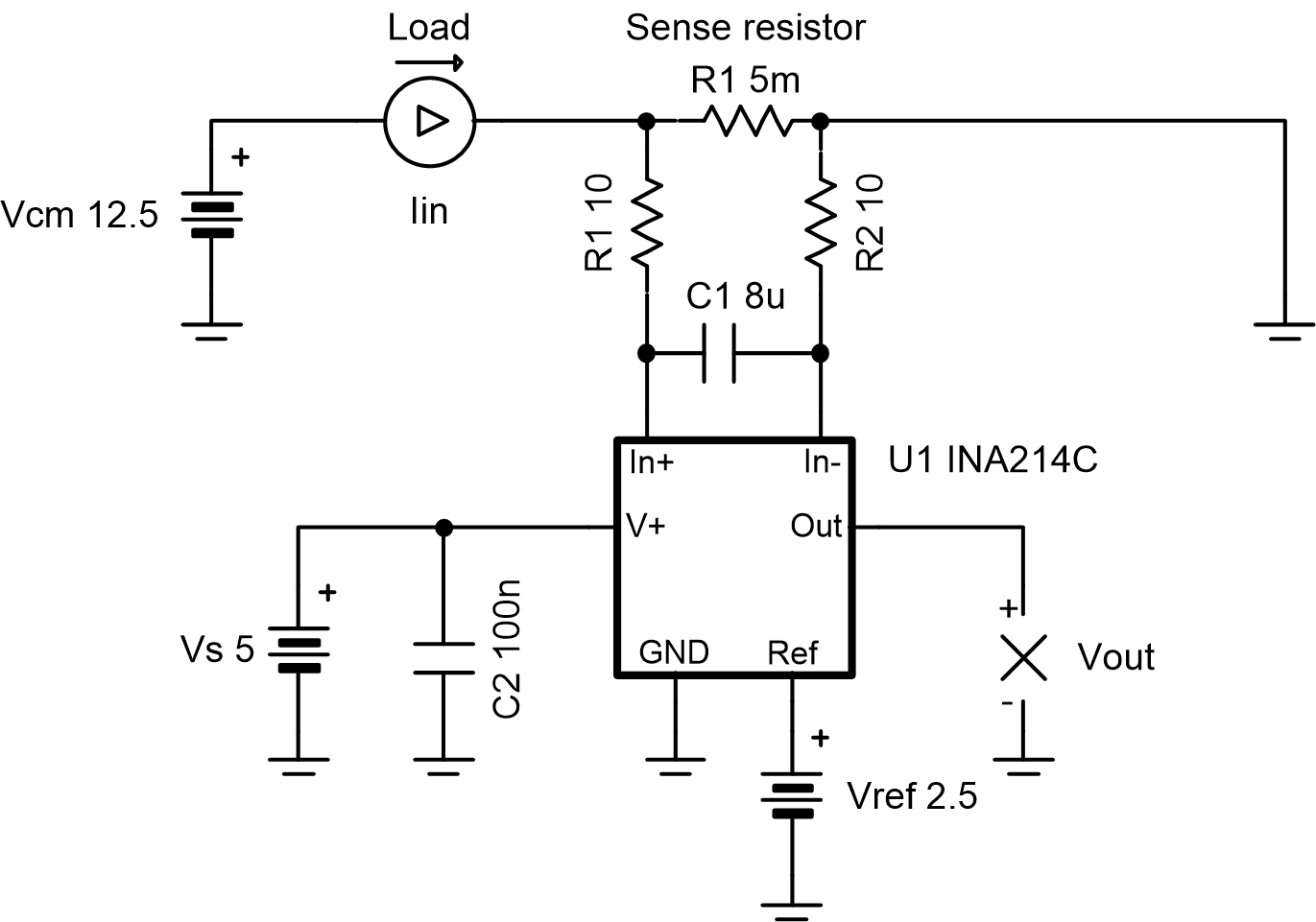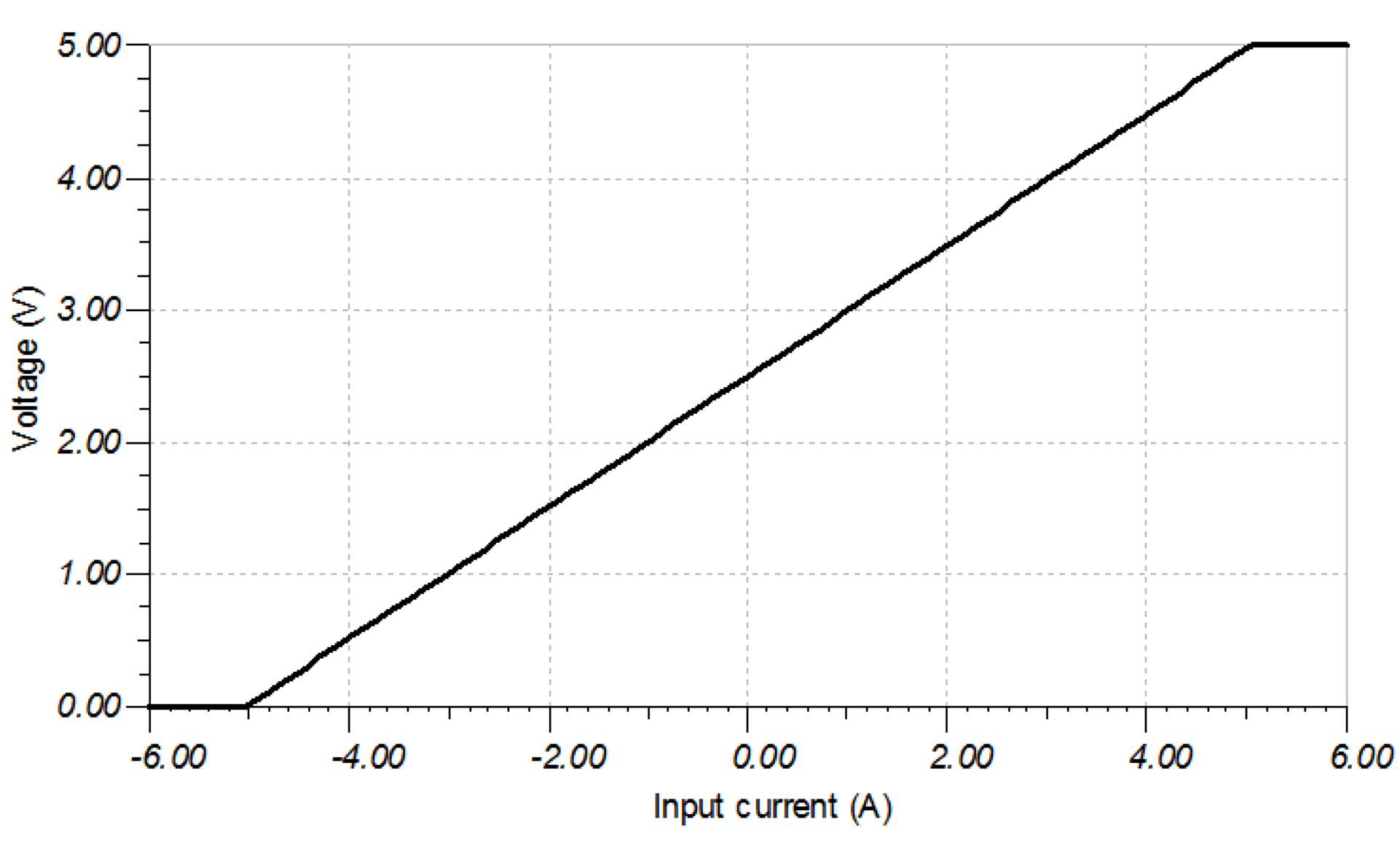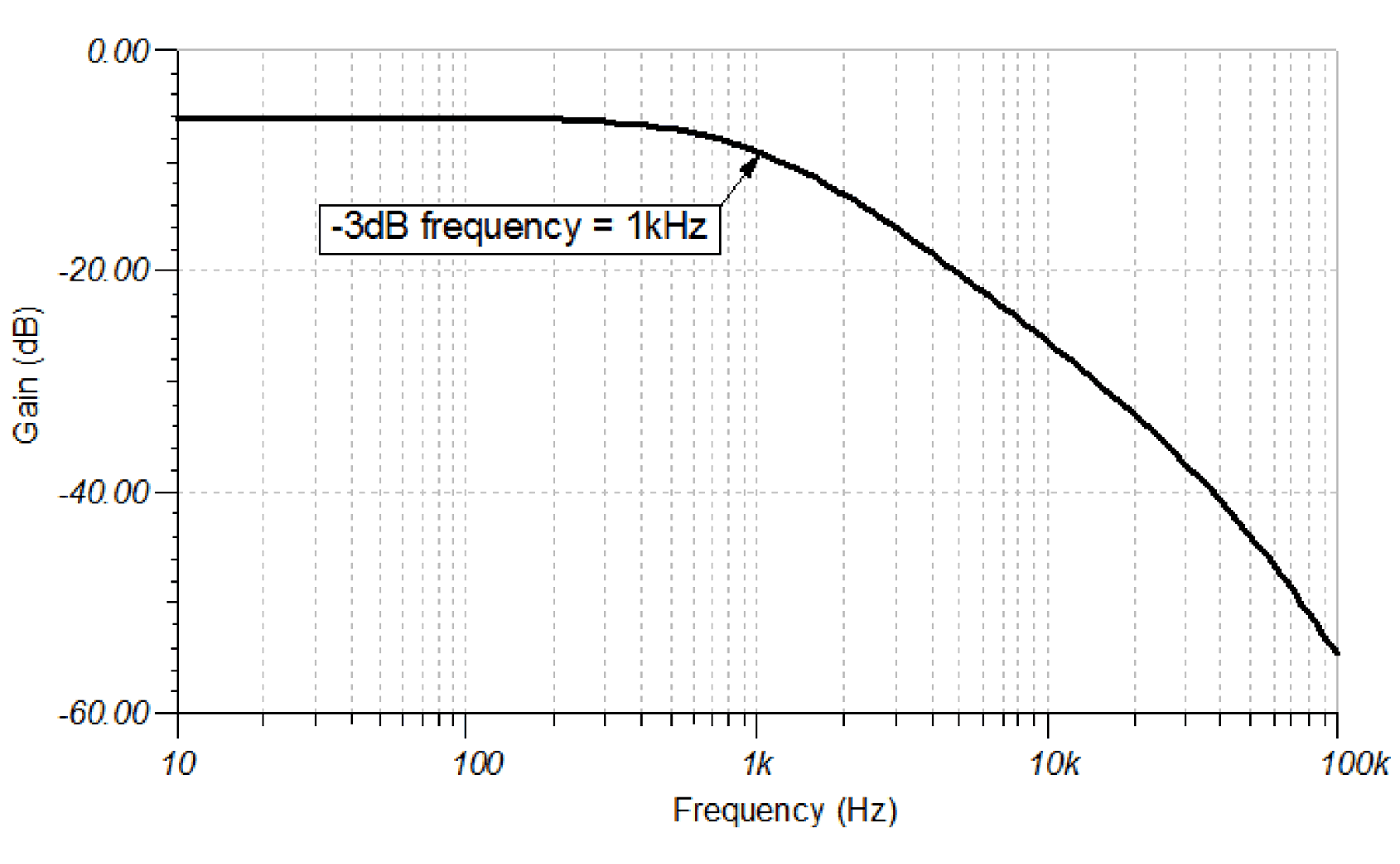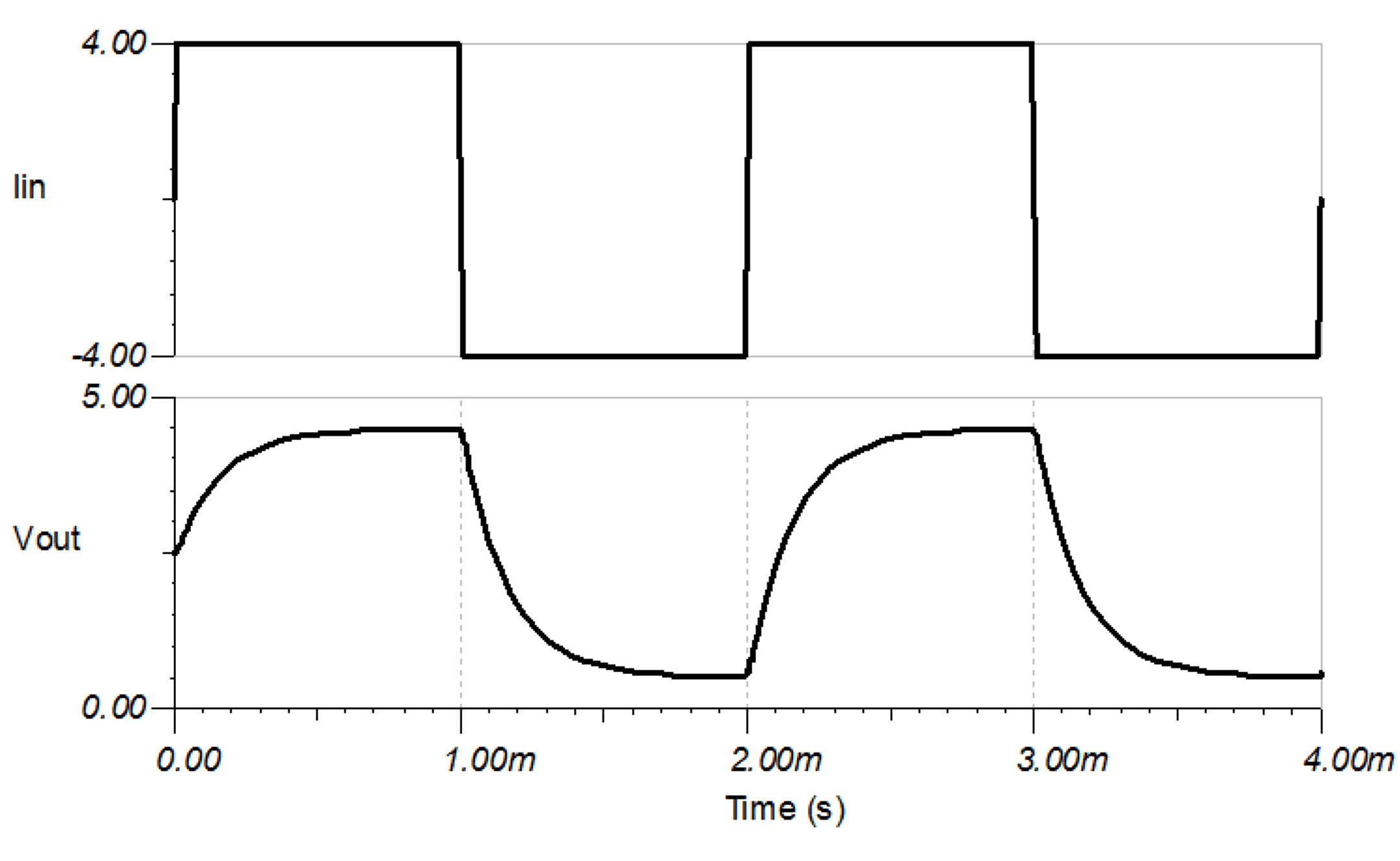SBOA300A June   2018  – December 2020

Design Goals

Input Output Supply
IinMin IinMax Vcm VoutMin VoutMax Vs Vref
–4A 4A 12.5 V 0.5 V 4.5 V 5 2.5 V

Design Description

The low-side bidirectional current-shunt monitor solution illustrated in the following image can accurately measure currents from –4A to 4A, and the design parameters can easily be changed for different current measurement ranges. Current-shunt monitors from the INA21x family have integrated precision gain resistors and a zero-drift architecture that enables current sensing with maximum drops across the shunt as low as 10mV full-scale.Design Notes

Design Steps

1. Determine Vref based on the desired current range:

With a current range of –4A to 4A, then half of the range is below 0V, so set:

Equation 1. ${V}_{ref}=\frac{1}{2}{V}_{s}=\frac{5}{2}=2.5V$
2. Determine the desired shunt resistance based on the maximum current and maximum output voltage:

To not exceed the swing-to-rail and to allow for some margin, use VoutMax = 4.5V. This, combined with maximum current of 4A and the Vref calculated in step 1, can be used to determine the shunt resistance using the equation:

Equation 1. ${R}_{1}=\frac{{V}_{\mathrm{outMax}}-{V}_{\mathrm{ref}}}{\mathrm{Gain}×{I}_{\mathrm{loadMax}}}=\frac{4.5-2.5}{100×4}=5\mathrm{m\Omega }$
3. Confirm Vout will be within the desired range:

At the maximum current of 4A, with Gain = 100V/V, R1 = 5mΩ, and Vref = 2.5V:

Equation 1. ${V}_{\mathrm{out}}={I}_{\mathrm{load}}×\mathrm{Gain}×{R}_{1}+{V}_{\mathrm{ref}}=4×100×0.005+2.5=4.5V$

At the minimum current of -4A, with Gain = 100V/V, R1 = 5mΩ, and Vref = 2.5V:

Equation 1. ${V}_{\mathrm{out}}={I}_{\mathrm{load}}×\mathrm{Gain}×{R}_{1}+{V}_{\mathrm{ref}}=-4×100×0.005+2.5=0.5V$
4. Filter cap selection:

To filter the input signal at 1kHz, using R1 = R2 = 10Ω:

Equation 1. ${C}_{1}=\frac{1}{2\pi \left({R}_{1}+{R}_{2}\right){F}_{-3\mathrm{dB}}}=\frac{1}{2\pi \left(10+10\right)1000}=7.958×{10}^{-6}\approx 8\mathrm{\mu F}$

For more information on signal filtering and the associated gain error, see INA21x Voltage Output, Low- or High-Side Measurement, Bidirectional, Zero-Drift Series, Current-Shunt Monitors.

Design Simulations

DC Analysis Simulation Results

The following plot shows the simulated output voltage Vout for the given input current Iin.AC Analysis Simulation Results

The following plot shows the simulated gain vs frequency, as designed for in the design steps.Transient Analysis Simulation Results

The following plot shows the simulated delay and settling time of the output Vout for a step response in Iin from –4A to 4A.